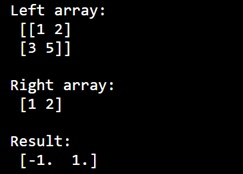# Solving Systems of Linear Equations with Python's NumPy

Learn, how to solve an array of linear equation systems with Python's NumPy?
Submitted by Pranit Sharma, on January 02, 2023

NumPy is an abbreviated form of Numerical Python. It is used for different types of scientific operations in python. Numpy is a vast library in python which is used for almost every kind of scientific or mathematical operation. It is itself an array which is a collection of various methods and functions for processing the arrays.

## NumPy - Solving Systems of Linear Equations

Suppose we are given an m×n array of linear equation systems (same LHS, different RHS).

It has the following features:

• one left-hand side: a single ndarray, shape (3, 3)
• m×n right-hand sides as one ndarray, shape (m, n, 3)

Then we would like m×n solutions, also as an (m, n, 3) ndarray.

For this purpose, we can use broadcasting to solve multiple systems of linear equations in a single call to numpy.linalg.solve

We will reshape the right-hand sides RHS to (3, m, n). This will make it so that the first dimension (index 0) corresponds to the variables in the system, the second dimension (index 1) corresponds to the different systems, and the third dimension (index 2) corresponds to the different right-hand sides for each system.

Let us understand with the help of an example,

## Python Code for Solving Systems of Linear Equations with Python's NumPy

```# Import numpy
import numpy as np

# Creating the left-hand side array
Left = np.array([[1, 2], [3, 5]])

# Creating the right-hand side array
Right = np.array([1, 2])

# Display original arrays
print("Left array:\n",Left,"\n")
print("Right array:\n",Right,"\n")

# Solving equation
res = np.linalg.solve(Left, Right)

# Display result
print("Result:\n",res)
```

Output: